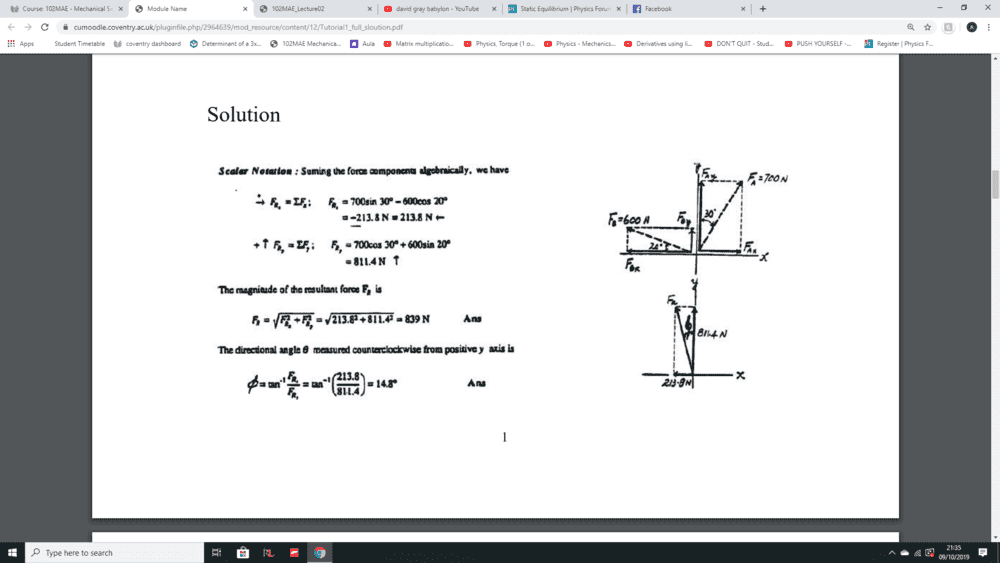# Static Equilibrium

• Engineering
Mason98
Homework Statement:
Sin for Y axis and Cos for X axis?
Relevant Equations:
Sin = opp/hyp Cos = Adj / hyp
When calculating static equilibrium, i know that you have to split for example, F1 into F1x and F1y. when using soh/cah/toa is it always SIN for the Y axis and always Cos for the X axis? I've been following this method and getting it wrong some times it's really stressing me out, Thanks for any help in advance.Last edited:

Mentor
when using soh/cah/toa is it always SIN for the Y axis and always Cos for the X axis?
No. It all depends on what angle you are using. If the angle is with respect to the x-axis, then fine: The x-component would use cosine, etc. But if the given angle is with respect to the y-axis, things are reversed.

Remember that sinθ = cos (90° - θ).

•Mason98
Mason98
so that is how in the picture Fax is sin because the angle given is in respect to the y-axis which would mean to use sin?

Mentor
so that is how in the picture Fax is sin because the angle given is in respect to the y-axis which would mean to use sin?
Exactly!

•Mason98
Mason98
Exactly!
Thank you very much! I appreciate your help :)

Staff Emeritus